## Simson Line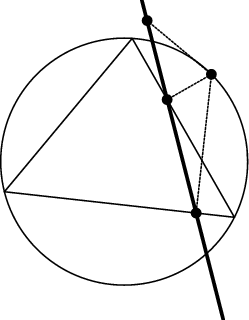The Simson line is the Line containing the feet of the perpendiculars from a point on the Circumcircle of a Triangle to the sides (or their extensions) of the Triangle. The Simson line is sometimes known as the Wallace-Simson Line, since it does not appear in any work of Simson (Johnson 1929, p. 137).

The Angle between the Simson lines of two points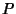and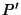is half the Angle of the arc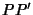. The Simson line of any Vertex is the Altitude through that Vertex. The Simson line of a point opposite a Vertex is the corresponding side. If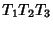is the Simson line of a point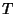of the Circumcircle, then the triangles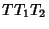and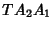are directly similar.